# Texas Go Math Grade 4 Lesson 4.2 Answer Key Compare Fractions

Refer to our Texas Go Math Grade 4 Answer Key Pdf to score good marks in the exams. Test yourself by practicing the problems from Texas Go Math Grade 4 Lesson 4.2 Answer Key Compare Fractions.

## Texas Go Math Grade 4 Lesson 4.2 Answer Key Compare Fractions

Essential Question

How con you compare fractions?
Explanation:
Use equivalent fractions with the same denominator.
When two fractions have the same denominator, they have equal-size parts. You can compare the number of parts.

Unlock the Problem

Every year, Avery’s school has a fair. This year, $$\frac{3}{8}$$ of the booths had face painting and $$\frac{1}{4}$$ of the booths had sand art. Were there more booths with face painting or sand art?
$$\frac{3}{8}$$ has more that means face painting has more

Compare $$\frac{3}{8}$$ and $$\frac{1}{4}$$.
$$\frac{3}{8}$$ has more that means face painting has more

One Way Use equivalent fractions with the same denominator.

When two fractions have the same denominator, they have equal-size parts. You can compare the number of parts.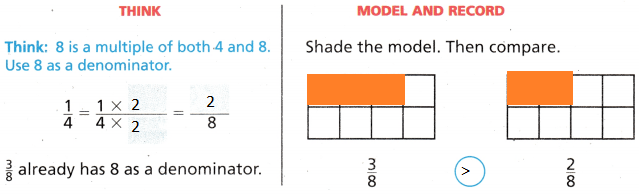Another Way Use equivalent fractions that have the same numerator.

When two fractions have the same numerator, they represent the same number of parts. You can compare the size of the parts.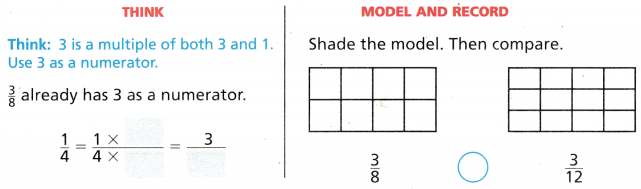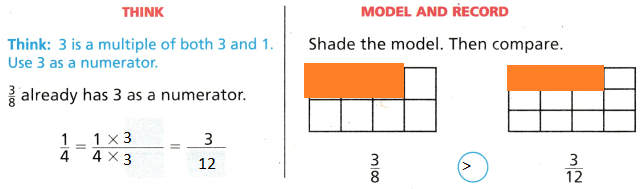Since $$\frac{3}{8}$$ _________ $$\frac{1}{4}$$, there were more booths with _________ .
Since $$\frac{3}{8}$$ > $$\frac{1}{4}$$, there were more booths with  . $$\frac{3}{8}$$

Share and Show

Question 1.
Compare $$\frac{2}{5}$$ and $$\frac{1}{10}$$.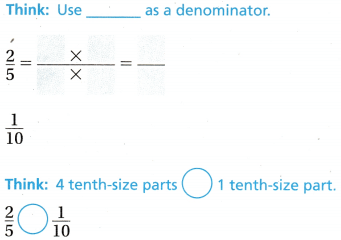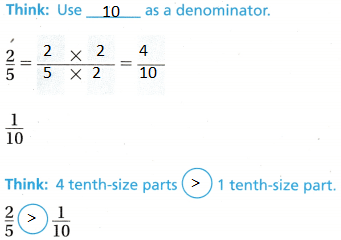Explanation:
We have to make the denominators same to know weather the fraction is greater or lesser

Question 2.
Compare $$\frac{6}{10}$$ and $$\frac{3}{4}$$.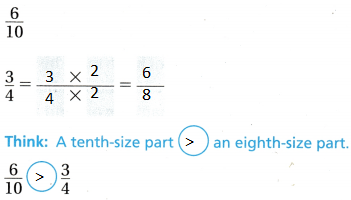Explanation:
We have to make the denominators same to know weather the fraction is greater or lesser

Compare. Write <, >, or =.

Question 3.
$$\frac{7}{8}$$ ___________ $$\frac{2}{8}$$
$$\frac{7}{8}$$ > $$\frac{2}{8}$$
Explanation:
We have to make the denominators same to know weather the fraction is greater or lesser
compared with the symbols

Question 4.
$$\frac{5}{12}$$ ___________ $$\frac{3}{6}$$
$$\frac{5}{12}$$ < $$\frac{3}{6}$$
Explanation:
We have to make the denominators same to know weather the fraction is greater or lesser
compared with the symbols
$$\frac{6}{12}$$ = $$\frac{3}{6}$$

Question 5.
$$\frac{4}{10}$$ ___________ $$\frac{4}{6}$$
$$\frac{4}{10}$$ < $$\frac{4}{6}$$
Explanation:
We have to make the denominators same to know weather the fraction is greater or lesser
compared with the symbols

Question 6.
$$\frac{6}{12}$$ ___________ $$\frac{2}{4}$$
$$\frac{6}{12}$$ = $$\frac{2}{4}$$
Explanation:
We have to make the denominators same to know weather the fraction is greater or lesser
compared with the symbols

Math Talk

MathematicaI Processes
Explain why using the same numerator or the same denominator can help you compare fractions.

Use equivalent fractions that have the same numerator.

When two fractions have the same numerator, they represent the same number of parts. You can compare the size of the parts.

Problem Solving

H.O.T. Algebra Find a number that makes statement true.

Question 7.
$$\frac{1}{2}$$ > $$\frac{}{3}$$
$$\frac{1}{2}$$ > $$\frac{1}{3}$$
Explanation:
The fractions are compared with $$\frac{1}{2}$$

Question 8.
$$\frac{3}{10}$$ > $$\frac{}{5}$$
$$\frac{3}{10}$$ > $$\frac{1}{5}$$
Explanation:
By making the denominators same and compared the shaded parts

Question 9.
$$\frac{5}{12}$$ > $$\frac{}{3}$$
$$\frac{5}{12}$$ > $$\frac{1}{3}$$
Explanation:
By making the denominators same and compared the shaded parts

Question 10.
$$\frac{2}{3}$$ > $$\frac{4}{}$$
$$\frac{2}{3}$$ > $$\frac{4}{12}$$
Explanation:
By making the denominators same and compared the shaded parts
$$\frac{4}{12}$$ = $$\frac{1}{3}$$

Question 11.
Multi-Step Lafayette has one book that weighs $$\frac{5}{8}$$ pound and another that weighs $$\frac{2}{3}$$ pound. Compare the weights using <, >, or =.
$$\frac{5}{8}$$ > $$\frac{2}{3}$$
Explanation:
By making the denominators equal and compared the fractions

Question 12.
Multi-Step Gena, Freddie, and Hank went running. Gena ran $$\frac{1}{3}$$ mile, Freddie ran $$\frac{4}{7}$$ mile, and Hank ran $$\frac{2}{3}$$ mile. Who ran the farthest? Explain your reasoning.
Hank ran more
Explanation:
By making the denominators equal compared the fractions

Problem Solving

Question 13.
H.O.T. Multi-Step Jerry is making a strawberry smoothie. Which statement about the recipe is true?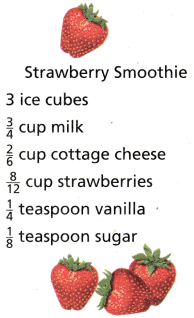(A) The amount of strawberries is greater than the amount of milk.
(B) The amount of milk is less than the amount of cottage cheese.
(C) The amount of strawberries is equal to the amount of cottage cheese.
(D) The amount of vanilla is greater than the amount of sugar.

a. What do you need to find?
We need find the amounts of weight of products

b. How will you find the answer?
By making the denominator equal

option A is corrcet

d. Fill in the bubble for the correct answer choice above.
Option A is bubbled

Question 14.
Multi-Step Mattie has $$\frac{3}{8}$$ pound of apples, $$\frac{7}{8}$$ pound of oranges, and $$\frac{3}{4}$$ pound of peaches. Which fruit weighs the least?
(A) They weigh the same
(B) oranges
(C) apples
(D) peaches
Explanation:
Apples weighs less than all the fruits

Question 15.
One kite reached a height of $$\frac{1}{4}$$ mile. The other kite reached a height of $$\frac{3}{16}$$ mile. What can you say about the two heights?
(A) $$\frac{1}{4}$$mile = $$\frac{3}{16}$$mile
(B) $$\frac{3}{16}$$mile > $$\frac{1}{4}$$mile
(C) $$\frac{1}{4}$$mile > $$\frac{3}{16}$$mile
(D) $$\frac{1}{4}$$mile < $$\frac{3}{16}$$mile
Explanation:
$$\frac{1}{4}$$mile > $$\frac{3}{16}$$mile say about the 2 heights

Question 16.
Apply Students decorated their piñatas with paint, flowers, feathers, and glitter. They used $$\frac{3}{4}$$ bottle of green glitter and $$\frac{5}{8}$$ bottle of silver glitter. Which shows how the amounts of green and silver glitter compare?
(A) $$\frac{3}{4}$$ < $$\frac{5}{8}$$
(B) $$\frac{3}{4}$$ > $$\frac{5}{8}$$
(C) $$\frac{5}{8}$$ < $$\frac{3}{4}$$
(D) $$\frac{3}{4}$$ = $$\frac{5}{8}$$
Explanation:
$$\frac{5}{8}$$ < $$\frac{3}{4}$$ shows that the amounts of green and silver glitter compare

Question 17.
Stephen ran $$\frac{1}{4}$$ mile and Tara ran $$\frac{2}{5}$$ mile. Which shows how $$\frac{2}{5}$$ and $$\frac{1}{4}$$ compare?
(A) $$\frac{1}{4}$$ = $$\frac{2}{5}$$
(B) $$\frac{2}{5}$$ > $$\frac{1}{4}$$
(C) $$\frac{2}{5}$$ < $$\frac{1}{4}$$
(D) $$\frac{1}{4}$$ > $$\frac{2}{5}$$
Explanation:
$$\frac{2}{5}$$ > $$\frac{1}{4}$$ compares $$\frac{2}{5}$$ and $$\frac{1}{4}$$

Question 18.
Multi-Step Students cut a pepperoni pizza into 12 equal slices and ate 5 slices. They cut a veggie pizza into 6 equal slices and ate 4 slices. Which statement compares the amounts of each pizza that were eaten?
(A) $$\frac{12}{5}$$ = $$\frac{6}{4}$$
(B) $$\frac{5}{17}$$ > $$\frac{4}{10}$$
(C) $$\frac{5}{12}$$ < $$\frac{4}{6}$$
(D) $$\frac{4}{6}$$ > $$\frac{5}{12}$$
Explanation:
$$\frac{4}{6}$$ > $$\frac{5}{12}$$ statement compares the amounts of each pizza that were eaten

TEXAS Test Prep

Question 19.
Simon studied $$\frac{7}{8}$$ hour, Marci studied $$\frac{7}{12}$$ hour, and John studied $$\frac{15}{16}$$ hour. Which one of the following comparisons is true?
(A) $$\frac{15}{16}$$ > $$\frac{7}{8}$$ and $$\frac{7}{8}$$ > $$\frac{7}{12}$$
(B) $$\frac{7}{12}$$ > $$\frac{7}{8}$$ and $$\frac{7}{8}$$ > $$\frac{15}{16}$$
(C) $$\frac{7}{12}$$ < $$\frac{7}{8}$$ and $$\frac{7}{8}$$ > $$\frac{15}{16}$$
(D) $$\frac{15}{16}$$ > $$\frac{7}{12}$$ and $$\frac{7}{12}$$ > $$\frac{7}{8}$$

### Texas Go Math Grade 4 Lesson 4.2 Homework and Practice Answer Key

Compare. Write <, >, or =.

Question 1.
$$\frac{1}{3}$$ __________ $$\frac{1}{4}$$
$$\frac{1}{3}$$ > $$\frac{1}{4}$$
Explanation:
We have to make the denominators same to know weather the fraction is greater or lesser
compared with the symbols

Question 2.
$$\frac{4}{5}$$ __________ $$\frac{8}{10}$$
$$\frac{4}{5}$$ = $$\frac{8}{10}$$
Explanation:
We have to make the denominators same to know weather the fraction is greater or lesser
compared with the symbols

Question 3.
$$\frac{3}{4}$$ __________ $$\frac{2}{4}$$
$$\frac{3}{4}$$ > $$\frac{2}{4}$$
Explanation:
We have to make the denominators same to know weather the fraction is greater or lesser
compared with the symbols

Question 4.
$$\frac{3}{10}$$ __________ $$\frac{2}{4}$$
$$\frac{3}{10}$$ < $$\frac{2}{4}$$
Explanation:
We have to make the denominators same to know weather the fraction is greater or lesser
compared with the symbols

Question 5.
$$\frac{75}{100}$$ __________ $$\frac{8}{10}$$
$$\frac{75}{100}$$ < $$\frac{8}{10}$$
Explanation:
We have to make the denominators same to know weather the fraction is greater or lesser
compared with the symbols

Question 6.
$$\frac{4}{6}$$ __________ $$\frac{2}{3}$$
$$\frac{4}{6}$$ < $$\frac{2}{3}$$
Explanation:
We have to make the denominators same to know weather the fraction is greater or lesser
compared with the symbols

Find a number that makes the statement true.

Question 7.
$$\frac{1}{2}$$ < $$\frac{}{8}$$
$$\frac{1}{2}$$ < $$\frac{1}{8}$$
Explanation:
By making the denominators same and compared the shaded parts

Question 8.
$$\frac{3}{10}$$ < $$\frac{}{20}$$
$$\frac{3}{10}$$ < $$\frac{8}{20}$$
Explanation:
By making the denominators same and compared the shaded parts

Question 9.
$$\frac{4}{5}$$ < $$\frac{2}{}$$
$$\frac{4}{5}$$ < $$\frac{2}{2}$$
Explanation:
By making the denominators same and compared the shaded parts

Question 10.
$$\frac{1}{2}$$ < $$\frac{5}{}$$
$$\frac{1}{2}$$ < $$\frac{5}{5}$$
Explanation:
By making the denominators same and compared the shaded parts

Question 11.
$$\frac{4}{5}$$ < $$\frac{}{10}$$
$$\frac{4}{5}$$ < $$\frac{10}{10}$$
Explanation:
By making the denominators same and compared the shaded parts

Question 12.
$$\frac{2}{3}$$ < $$\frac{3}{}$$
$$\frac{2}{3}$$ < $$\frac{3}{3}$$
Explanation:
By making the denominators same and compared the shaded parts

Problem Solving

Question 13.
At the yard sale, $$\frac{3}{4}$$ of the items for sale were toys and $$\frac{5}{8}$$ of the items for sale were books. Were there more toys or books for sale? Explain.
toys are more for sale
Explanation:
$$\frac{3}{4}$$ is multiplied with 2 to make the denominator equal
$$\frac{6}{8}$$ > $$\frac{5}{8}$$

Question 14.
A smoothie recipe calls for $$\frac{3}{4}$$ cup of milk and $$\frac{2}{3}$$ cup of yogurt. Does the recipe call for more milk or yogurt? Explain.
$$\frac{9}{12}$$ > $$\frac{8}{12}$$
Explanation:
$$\frac{3}{4}$$ multiplied with 3 $$\frac{9}{12}$$
$$\frac{2}{3}$$ multiplied with 4 $$\frac{8}{12}$$
so, milk is more

Question 15.
A puppy weighs $$\frac{7}{18}$$ pound and a kitten weighs $$\frac{4}{9}$$ pound. Which weighs more? Explain.
$$\frac{7}{18}$$ < $$\frac{8}{18}$$
Explanation:
Kitten weighs more
$$\frac{4}{9}$$ is multiplied with 2 to get the same denominator
and then compared

Question 16.
Tully ran $$\frac{7}{10}$$ mile and Maggie ran $$\frac{3}{5}$$ mile. Who ran farther? Explain.
By making the denominators equal
compared the numerators
Explanation:
Tully ran farther
$$\frac{3}{5}$$ multiplied with 2
to get the denominator 10
$$\frac{6}{10}$$

Lesson Check

Question 17.
Which number makes this statement true?
$$\frac{2}{7}$$ > $$\frac{}{5}$$
(A) 2
(B) 3
(C) 4
(D) 1
Explanation:
$$\frac{2}{7}$$ > $$\frac{1}{5}$$

Question 18.
The Garcia family ate $$\frac{8}{12}$$ of a pizza. What is $$\frac{8}{12}$$ in simplest form?
(A) $$\frac{2}{3}$$
(B) $$\frac{8}{12}$$
(C) $$\frac{1}{4}$$
(D) $$\frac{4}{6}$$
Explanation:
$$\frac{8}{12}$$ simplest form is $$\frac{2}{3}$$

Question 19.
Jarnie mixed $$\frac{2}{3}$$ bottle of cranberry juice and $$\frac{5}{6}$$ bottle of orange juice. Which shows how to compare $$\frac{2}{3}$$ and $$\frac{5}{6}$$ ?
(A) $$\frac{2}{3}$$ < $$\frac{5}{6}$$
(B) $$\frac{5}{6}$$ < $$\frac{2}{3}$$
(C) $$\frac{5}{6}$$ = $$\frac{2}{3}$$
(D) $$\frac{2}{3}$$ > $$\frac{5}{6}$$
Explanation:
$$\frac{2}{3}$$ < $$\frac{5}{6}$$ shows to compare $$\frac{2}{3}$$ < $$\frac{5}{6}$$

Question 20.
Stephan practiced $$\frac{9}{10}$$ hour on Saturday and $$\frac{3}{4}$$ hour on Sunday. Which statement compares $$\frac{9}{10}$$ and $$\frac{3}{4}$$?
(A) $$\frac{3}{4}$$ = $$\frac{9}{10}$$
(B) $$\frac{3}{4}$$ > $$\frac{9}{10}$$
(C) $$\frac{9}{10}$$ = $$\frac{3}{4}$$
(D) $$\frac{9}{10}$$ = $$\frac{3}{4}$$
Explanation:
$$\frac{3}{4}$$ > $$\frac{9}{10}$$

Question 21.
Multi-Step Angie, Blake, Carlos, and Daisy went running. Angie ran $$\frac{1}{3}$$ mile, Blake ran $$\frac{3}{5}$$ mile, Carlos ran $$\frac{7}{10}$$ mile, and Daisy ran $$\frac{1}{2}$$ mile. Who ran the farthest?
(A) Angie
(B) Blake
(C) Carlos
(D) Daisy
Explanation:
Carlos ran farther

Question 22.
Carmen is hanging framed pictures on her wall. One picture weighs $$\frac{4}{7}$$ pound and another weighs $$\frac{3}{4}$$ pound. Which statement correctly compares the weights?
(A) $$\frac{8}{14}$$ = $$\frac{3}{4}$$
(B) $$\frac{4}{7}$$ < $$\frac{3}{4}$$
(C) $$\frac{8}{14}$$ > $$\frac{3}{4}$$
(D) $$\frac{4}{7}$$ > $$\frac{3}{4}$$
$$\frac{4}{7}$$ < $$\frac{3}{4}$$ makes the statement statement correctly compares the weights.